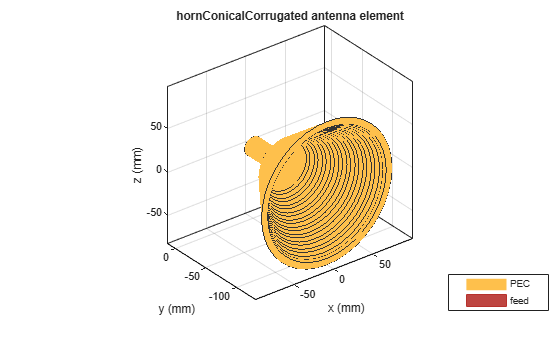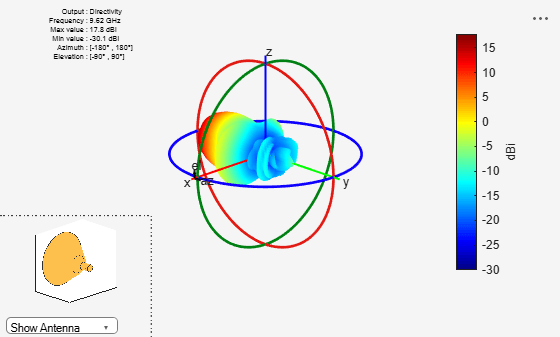# hornConicalCorrugated

Create conical corrugated-horn antenna

Since R2020a

## Description

The `hornConicalCorrugated` object creates a conical corrugated-horn antenna, with grooves covering the inner surface of the cone. These antennas are widely used as feed horns for dish reflector antennas as they have smaller side lobes and low cross-polarization level.## Creation

### Syntax

``ant = hornConicalCorrugated``
``ant = hornConicalCorrugated(Name,Value)``

### Description

````ant = hornConicalCorrugated` creates a corrugated conical-horn antenna object with default dimensions for an operating frequency around 9.5 GHz.```

example

````ant = hornConicalCorrugated(Name,Value)` sets properties using one or more name-value pairs. For example, `ant = hornConicalCorrugated('Radius',1)`, creates a conical corrugated-horn antenna with a radius of 1 meter.```

## Properties

expand all

Radius of the waveguide, specified as a real-valued scalar in meters.

Example: `'Radius',0.760`

Example: `ant.Radius = 0.760`

Data Types: `double`

Height of the waveguide, specified as a real-valued scalar in meters.

Example: `'WaveguideHeight',0.0340`

Example: `ant.WaveguideHeight = 0.0340`

Data Types: `double`

Height of the feed, specified as a real-valued scalar in meters.

Example: `'FeedHeight',0.0085`

Example: `ant.FeedHeight = 0.0085`

Data Types: `double`

Width of the feed, specified as a real-valued scalar in meters.

Example: `'FeedWidth',0.0200`

Example: `ant.FeedWidth = 0.0200`

Data Types: `double`

Signed distance of the feed along the y-axis, specified as a real-valued scalar in meters.

Example: `'FeedOffset',0.03627`

Example: `ant.FeedOffset = 0.3627`

Data Types: `double`

Height of the cone, specified as a real-valued scalar in meters.

Example: `'ConeHeight',0.0540`

Example: `ant.ConeHeight = 0.0540`

Data Types: `double`

Radius of the cone aperture, specified as a real-valued scalar in meters.

Example: `'ApertureRadius',0.0560`

Example: `ant.ApertureRadius = 0.0790`

Data Types: `double`

Distance between two successive corrugations, specified as a real-valued scalar in meters.

Example: `'Pitch',0.0060`

Example: `ant.Pitch = 0.0090`

Data Types: `double`

Distance of first corrugation from waveguide, specified as a real-valued scalar in meters.

Example: `'FirstCorrugatedDistance',0.0360`

Example: ```ant.FirstCorrugatedDistance = 0.0190```

Data Types: `double`

Corrugation width, specified as a real-valued scalar in meters.

Example: `'CorrugateWidth',0.0058`

Example: `ant.CorrugateWidth = 0.0019`

Data Types: `double`

Corrugation depth, specified as a real-valued scalar in meters.

Example: `'CorrugateDepth',0.0560`

Example: `ant.CorrugateDepth = 0.0790`

Data Types: `double`

Type of the metal used as a conductor, specified as a metal material object. You can choose any metal from the `MetalCatalog` or specify a metal of your choice. For more information, see `metal`. For more information on metal conductor meshing, see Meshing.

Example: ```m = metal('Copper'); 'Conductor',m```

Example: ```m = metal('Copper'); ant.Conductor = m```

Lumped elements added to the antenna feed, specified as a lumped element object. You can add a load anywhere on the surface of the antenna. By default, the load is at the feed. For more information, see `lumpedElement`.

Example: `'Load',lumpedelement`. `lumpedelement` is the object for the load created using `lumpedElement`.

Example: ```ant.Load = lumpedElement('Impedance',75)```

Tilt angle of the antenna, specified as a scalar or vector with each element unit in degrees. For more information, see Rotate Antennas and Arrays.

Example: `Tilt=90`

Example: `Tilt=[90 90]`,`TiltAxis=[0 1 0;0 1 1]` tilts the antenna at 90 degrees about the two axes defined by the vectors.

Note

The `wireStack` antenna object only accepts the dot method to change its properties.

Data Types: `double`

Tilt axis of the antenna, specified as:

• Three-element vector of Cartesian coordinates in meters. In this case, each coordinate in the vector starts at the origin and lies along the specified points on the X-, Y-, and Z-axes.

• Two points in space, each specified as three-element vectors of Cartesian coordinates. In this case, the antenna rotates around the line joining the two points in space.

• A string input describing simple rotations around one of the principal axes, 'X', 'Y', or 'Z'.

Example: `TiltAxis=[0 1 0]`

Example: `TiltAxis=[0 0 0;0 1 0]`

Example: `TiltAxis = 'Z'`

Data Types: `double`

## Object Functions

 `show` Display antenna, array structures or shapes `axialRatio` Axial ratio of antenna `beamwidth` Beamwidth of antenna `charge` Charge distribution on antenna or array surface `current` Current distribution on antenna or array surface `design` Design prototype antenna or arrays for resonance around specified frequency `efficiency` Radiation efficiency of antenna `EHfields` Electric and magnetic fields of antennas; Embedded electric and magnetic fields of antenna element in arrays `impedance` Input impedance of antenna; scan impedance of array `mesh` Mesh properties of metal, dielectric antenna, or array structure `meshconfig` Change mesh mode of antenna structure `optimize` Optimize antenna or array using SADEA optimizer `pattern` Radiation pattern and phase of antenna or array; Embedded pattern of antenna element in array `patternAzimuth` Azimuth pattern of antenna or array `patternElevation` Elevation pattern of antenna or array `rcs` Calculate and plot radar cross section (RCS) of platform, antenna, or array `returnLoss` Return loss of antenna; scan return loss of array `sparameters` Calculate S-parameter for antenna and antenna array objects `vswr` Voltage standing wave ratio of antenna

## Examples

collapse all

Create a conical corrugated-horn antenna object with the cone height set to 0.09 m

```ant = hornConicalCorrugated('ConeHeight',0.09); show(ant)```Plot the radiation pattern of the antenna at 9.62 GHz.

```figure pattern(ant,9.62e9)```Jadhav, Rohini.P, Vinothkurnar Javnrakash Dongre, Arunkumar Heddallikar. "Design of X-Band Conical Horn Antenna Using Coaxial Feed and Improved Design Technique for Bandwidth Enhancement". In International Conference on Computing, Communication, Control, and Automation (ICCUBEA), 1-6. Pune, India: ICCUBEA 2017.